Скачать презентацию Goal of the Lecture Understand how much a

42578326613491c357bcffb921511f4a.ppt

• Количество слайдов: 11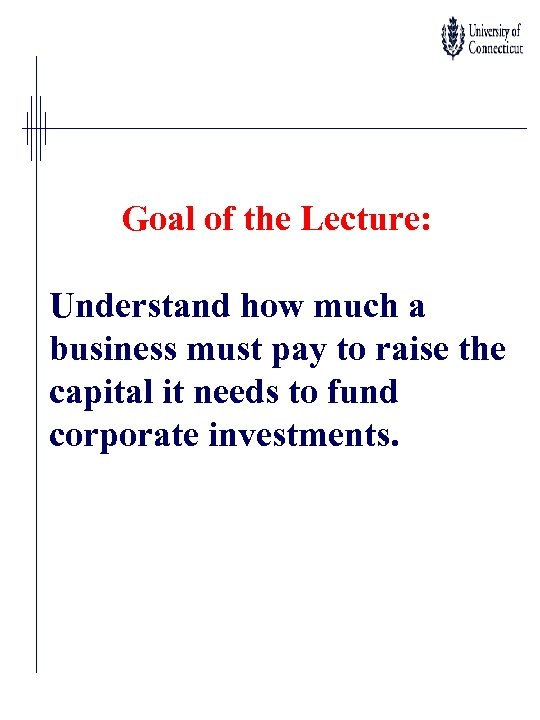Goal of the Lecture: Understand how much a business must pay to raise the capital it needs to fund corporate investments.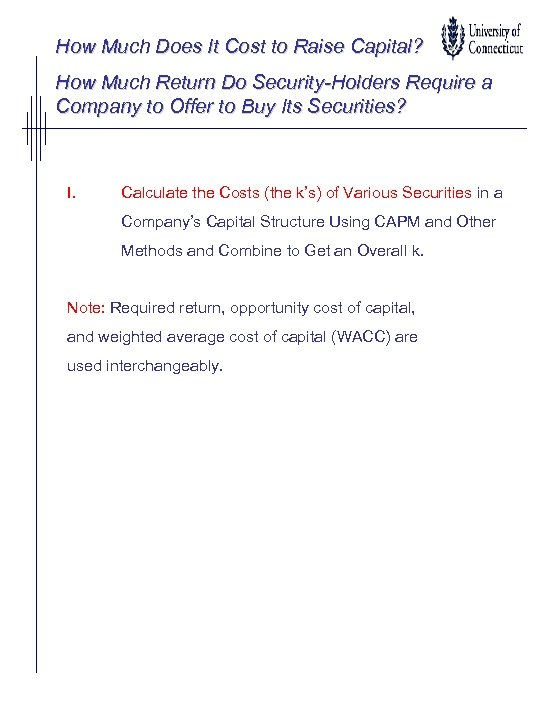How Much Does It Cost to Raise Capital? How Much Return Do Security-Holders Require a Company to Offer to Buy Its Securities? I. Calculate the Costs (the k’s) of Various Securities in a Company’s Capital Structure Using CAPM and Other Methods and Combine to Get an Overall k. Note: Required return, opportunity cost of capital, and weighted average cost of capital (WACC) are used interchangeably.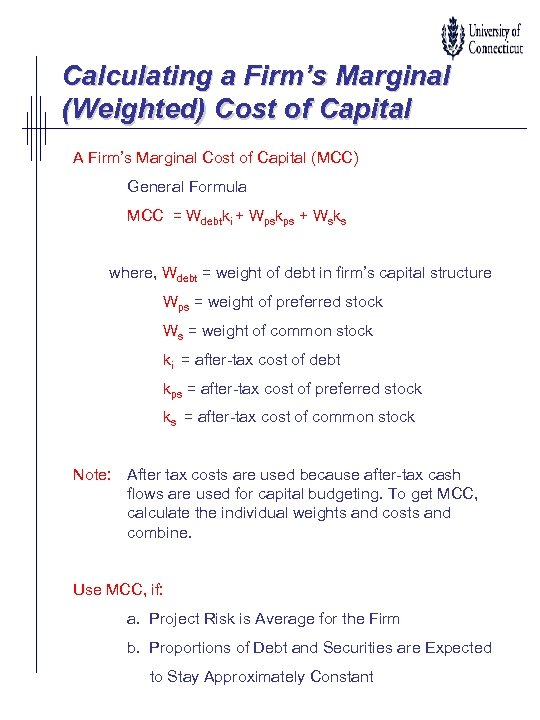Calculating a Firm’s Marginal (Weighted) Cost of Capital A Firm’s Marginal Cost of Capital (MCC) General Formula MCC = Wdebtki + Wpskps + Wsks where, Wdebt = weight of debt in firm’s capital structure Wps = weight of preferred stock Ws = weight of common stock ki = after-tax cost of debt kps = after-tax cost of preferred stock ks = after-tax cost of common stock Note: After tax costs are used because after-tax cash flows are used for capital budgeting. To get MCC, calculate the individual weights and costs and combine. Use MCC, if: a. Project Risk is Average for the Firm b. Proportions of Debt and Securities are Expected to Stay Approximately Constant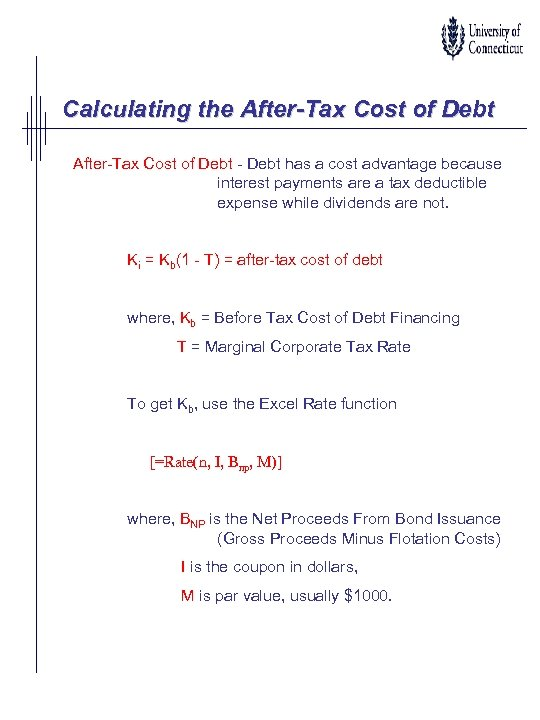Calculating the After-Tax Cost of Debt - Debt has a cost advantage because interest payments are a tax deductible expense while dividends are not. Ki = Kb(1 - T) = after-tax cost of debt where, Kb = Before Tax Cost of Debt Financing T = Marginal Corporate Tax Rate To get Kb, use the Excel Rate function [=Rate(n, I, Bnp, M)] where, BNP is the Net Proceeds From Bond Issuance (Gross Proceeds Minus Flotation Costs) I is the coupon in dollars, M is par value, usually \$1000.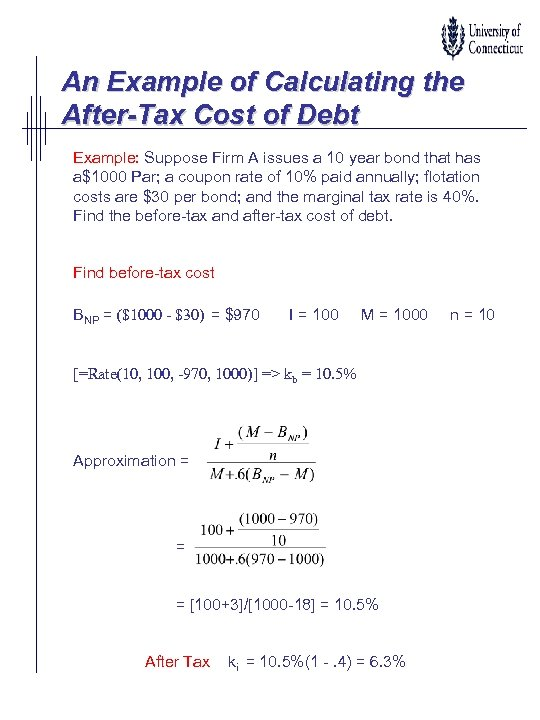An Example of Calculating the After-Tax Cost of Debt Example: Suppose Firm A issues a 10 year bond that has a\$1000 Par; a coupon rate of 10% paid annually; flotation costs are \$30 per bond; and the marginal tax rate is 40%. Find the before-tax and after-tax cost of debt. Find before-tax cost BNP = (\$1000 - \$30) = \$970 I = 100 M = 1000 [=Rate(10, 100, -970, 1000)] => kb = 10. 5% Approximation = = = [100+3]/[1000 -18] = 10. 5% After Tax ki = 10. 5%(1 -. 4) = 6. 3% n = 10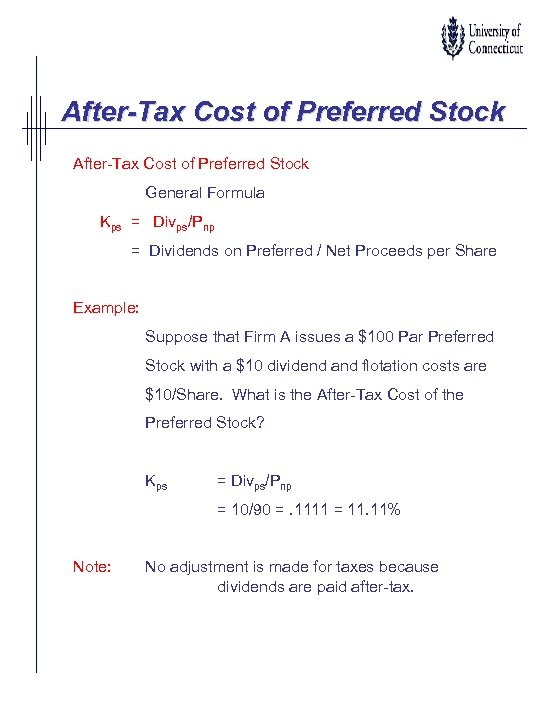After-Tax Cost of Preferred Stock General Formula Kps = Divps/Pnp = Dividends on Preferred / Net Proceeds per Share Example: Suppose that Firm A issues a \$100 Par Preferred Stock with a \$10 dividend and flotation costs are \$10/Share. What is the After-Tax Cost of the Preferred Stock? Kps = Divps/Pnp = 10/90 =. 1111 = 11. 11% Note: No adjustment is made for taxes because dividends are paid after-tax.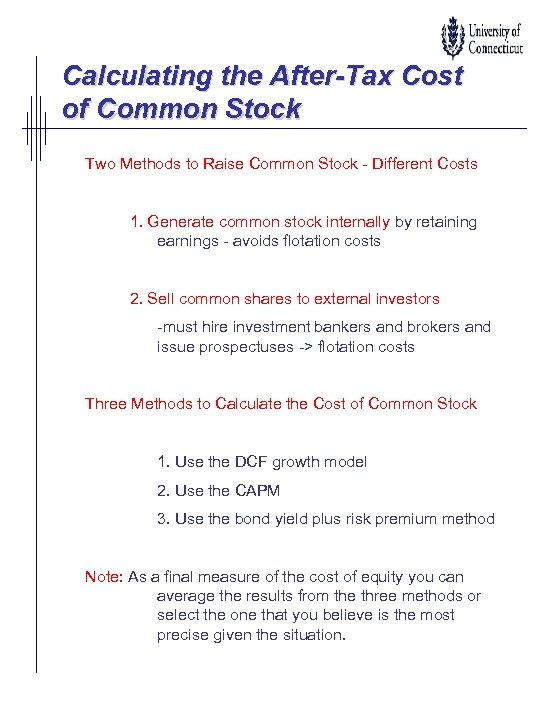Calculating the After-Tax Cost of Common Stock Two Methods to Raise Common Stock - Different Costs 1. Generate common stock internally by retaining earnings - avoids flotation costs 2. Sell common shares to external investors -must hire investment bankers and brokers and issue prospectuses -> flotation costs Three Methods to Calculate the Cost of Common Stock 1. Use the DCF growth model 2. Use the CAPM 3. Use the bond yield plus risk premium method Note: As a final measure of the cost of equity you can average the results from the three methods or select the one that you believe is the most precise given the situation.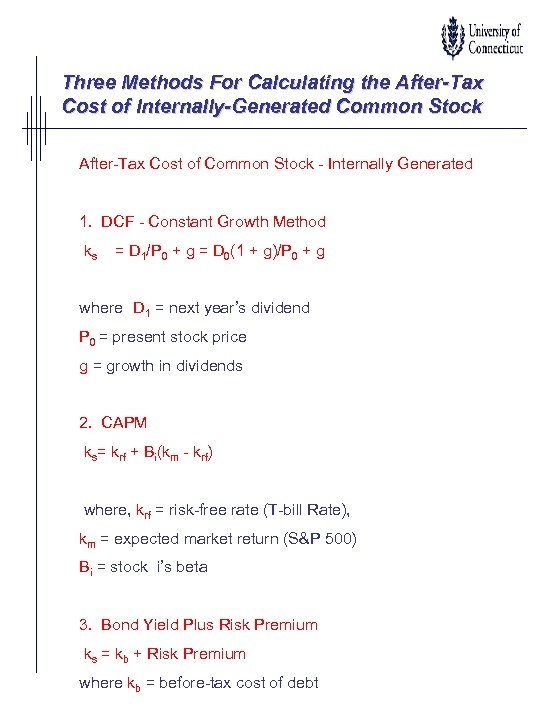Three Methods For Calculating the After-Tax Cost of Internally-Generated Common Stock After-Tax Cost of Common Stock - Internally Generated 1. DCF - Constant Growth Method ks = D 1/P 0 + g = D 0(1 + g)/P 0 + g where D 1 = next year’s dividend P 0 = present stock price g = growth in dividends 2. CAPM ks= krf + Bi(km - krf) where, krf = risk-free rate (T-bill Rate), km = expected market return (S&P 500) Bi = stock i’s beta 3. Bond Yield Plus Risk Premium ks = kb + Risk Premium where kb = before-tax cost of debt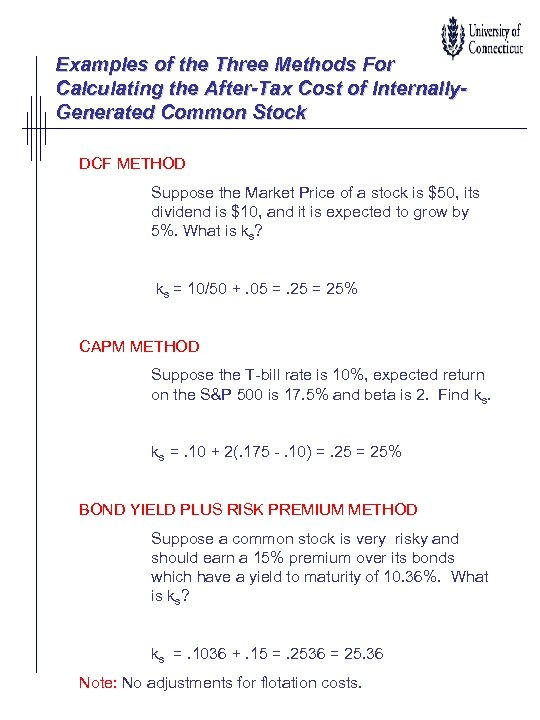Examples of the Three Methods For Calculating the After-Tax Cost of Internally. Generated Common Stock DCF METHOD Suppose the Market Price of a stock is \$50, its dividend is \$10, and it is expected to grow by 5%. What is ks? ks = 10/50 +. 05 =. 25 = 25% CAPM METHOD Suppose the T-bill rate is 10%, expected return on the S&P 500 is 17. 5% and beta is 2. Find ks. ks =. 10 + 2(. 175 -. 10) =. 25 = 25% BOND YIELD PLUS RISK PREMIUM METHOD Suppose a common stock is very risky and should earn a 15% premium over its bonds which have a yield to maturity of 10. 36%. What is ks? ks =. 1036 +. 15 =. 2536 = 25. 36 Note: No adjustments for flotation costs.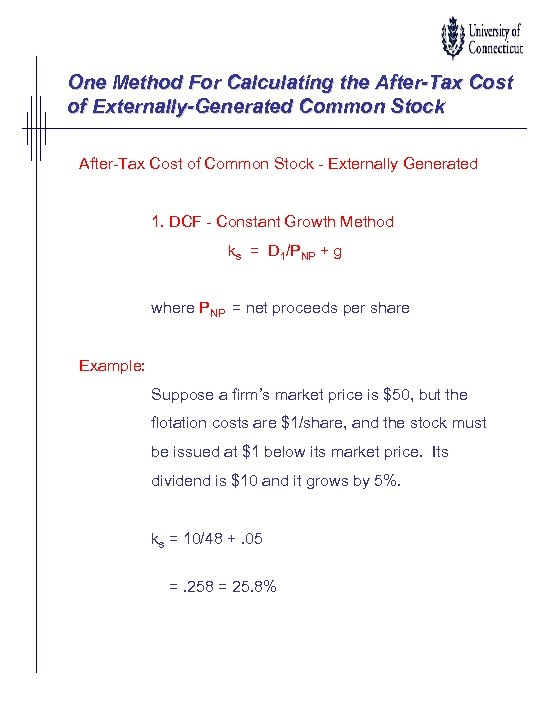One Method For Calculating the After-Tax Cost of Externally-Generated Common Stock After-Tax Cost of Common Stock - Externally Generated 1. DCF - Constant Growth Method ks = D 1/PNP + g where PNP = net proceeds per share Example: Suppose a firm’s market price is \$50, but the flotation costs are \$1/share, and the stock must be issued at \$1 below its market price. Its dividend is \$10 and it grows by 5%. ks = 10/48 +. 05 =. 258 = 25. 8%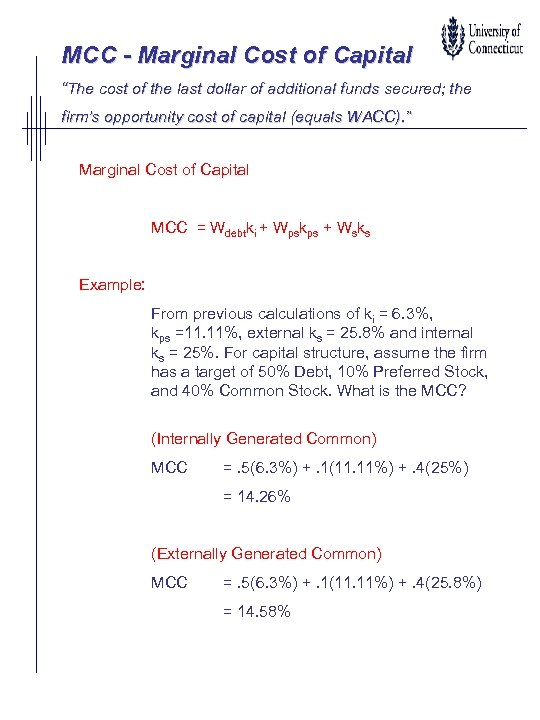MCC - Marginal Cost of Capital “The cost of the last dollar of additional funds secured; the firm’s opportunity cost of capital (equals WACC). ” Marginal Cost of Capital MCC = Wdebtki + Wpskps + Wsks Example: From previous calculations of ki = 6. 3%, kps =11. 11%, external ks = 25. 8% and internal ks = 25%. For capital structure, assume the firm has a target of 50% Debt, 10% Preferred Stock, and 40% Common Stock. What is the MCC? (Internally Generated Common) MCC =. 5(6. 3%) +. 1(11. 11%) +. 4(25%) = 14. 26% (Externally Generated Common) MCC =. 5(6. 3%) +. 1(11. 11%) +. 4(25. 8%) = 14. 58%